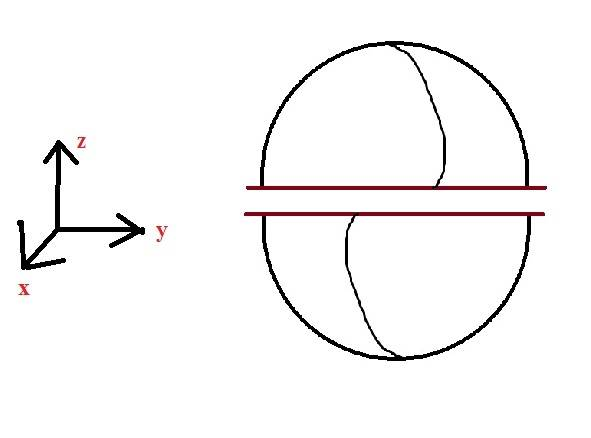# Force of magnetic attraction between hemispheres

Pouyan

## Homework Statement

Calculate the force of magnetic attraction between the northern and southern hemispheres of a uniformly charged spinning spherical shell, with radius R, angular velocity ω and surface charge density σ.

## Homework Equations

Maxwell Tensor : Tm = [/B](1/μ) * ((B*n)B - (1/2)*B2 n)

B_in =
(2/3)*μσRω*z
B_out =
μm/(4πr3) * (2cosθ r + sinθ θ)
where
m = (4/3)*πR3(σωR)

## The Attempt at a Solution

I do see in my solution that :
1-We use a surface consisting of the entire equatorial plane, CLOSING IT with a hemispherical surface at infinity where (since the field is zero out there) contribution is zero.
for r>R :
B = μm/(4πr3) θ = - μm/(4πr3) z (Why ?! )
2-
We have a equatorial circular disk. We use B inside and da = rdrdφ. Direction is in z-axis!
I don't want the solution because I already have it but MY QUESTIONS are :

A) How should think and imagine this spheres ?!
Should I think like this and use those formulas :B) Why we do write B = μm/(4πr3) θ = - μm/(4πr3) z
Why is θ =-z in this case ?! I don't get it !

I know the rest. That is just integrating

#### Attachments

Homework Helper
Gold Member
A) How should think and imagine this spheres ?!
Should I think like this and use those formulas :
I'm not quite following what you are asking here. Can you reword the question?

B) Why we do write B = μm/(4πr3) θ = - μm/(4πr3) z
Why is θ =-z in this case ?! I don't get it !

It is because you are using spherical coordinates with θ measured from the positive z axis. The unit vector θ points in the direction of increasing θ. For a point on the equatorial plane of the sphere, what is the direction of θ?

•Pouyan
Pouyan
I'm not quite following what you are asking here. Can you reword the question?

It is because you are using spherical coordinates with θ measured from the positive z axis. The unit vector θ points in the direction of increasing θ. For a point on the equatorial plane of the sphere, what is the direction of θ?
I've got it !

Thanks